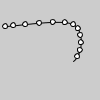Name

### curvePoint()

Examples```noFill()
curve(5, 26, 5, 26, 73, 24, 73, 61)
curve(5, 26, 73, 24, 73, 61, 15, 65)
fill(255)
ellipseMode(CENTER)
steps = 6
for i in range(steps):
t = i / float(steps)
x = curvePoint(5, 5, 73, 73, t)
y = curvePoint(26, 26, 24, 61, t)
ellipse(x, y, 5, 5)
x = curvePoint(5, 73, 73, 15, t)
y = curvePoint(26, 24, 61, 65, t)
ellipse(x, y, 5, 5)
```
Description Evaluates the curve at point t for points a, b, c, d. The parameter t may range from 0 (the start of the curve) and 1 (the end of the curve). a and d are points on the curve, and b and c are the control points. This can be used once with the x coordinates and a second time with the y coordinates to get the location of a curve at t.
Syntax
```curvePoint(a, b, c, d, t)
```
Parameters
a float: coordinate of first point on the curve float: coordinate of second point on the curve float: coordinate of third point on the curve float: coordinate of fourth point on the curve float: value between 0 and 1
Related curve()
curveVertex()
bezierPoint()

Updated on Mon Sep 21 15:53:24 2020.

If you see any errors or have comments, please let us know.